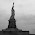## Sunday, April 1, 2012

### Simple Queue Data Structure in ANSI C

Introduction

This post is about how to implement a queue in c, can push item to tail, pop item from head, peek item without delete from head, display all items and get its size.

The program

Queue.c

``````
#include <stdio.h>
#include <stdlib.h>
/**
* This sample is about how to implement a queue in c
*
* Type of item is int
* Can get the size
* Can display all content
*/
/**
* The Node struct,
* contains item and the pointer that point to next node.
*/
typedef struct Node {
int item;
struct Node* next;
} Node;
/**
* The Queue struct, contains the pointers that
* point to first node and last node, the size of the Queue,
* and the function pointers.
*/
typedef struct Queue {
Node* tail;

void (*push) (struct Queue*, int); // add item to tail
// get item from head and remove it from queue
int (*pop) (struct Queue*);
// get item from head but keep it in queue
int (*peek) (struct Queue*);
// display all element in queue
void (*display) (struct Queue*);
// size of this queue
int size;
} Queue;
/**
* Push an item into queue, if this is the first item,
* both queue->head and queue->tail will point to it,
* otherwise the oldtail->next and tail will point to it.
*/
void push (Queue* queue, int item);
/**
* Return and remove the first item.
*/
int pop (Queue* queue);
/**
* Return but not remove the first item.
*/
int peek (Queue* queue);
/**
* Show all items in queue.
*/
void display (Queue* queue);
/**
* Create and initiate a Queue
*/
Queue createQueue ();
int main () {
Queue queue = createQueue();
queue.display(&queue);

printf("push item 2\n");
queue.push(&queue, 2);
printf("push item 3\n");
queue.push(&queue, 3);
printf("push item 6\n");
queue.push(&queue, 6);

queue.display(&queue);

printf("peek item %d\n", queue.peek(&queue));
queue.display(&queue);

printf("pop item %d\n", queue.pop(&queue));
printf("pop item %d\n", queue.pop(&queue));
queue.display(&queue);

printf("pop item %d\n", queue.pop(&queue));
queue.display(&queue);
printf("push item 6\n");
queue.push(&queue, 6);

queue.display(&queue);
system("PAUSE");
}

/**
* Push an item into queue, if this is the first item,
* both queue->head and queue->tail will point to it,
* otherwise the oldtail->next and tail will point to it.
*/
void push (Queue* queue, int item) {
// Create a new node
Node* n = (Node*) malloc (sizeof(Node));
n->item = item;
n->next = NULL;

} else{
queue->tail->next = n;
}
queue->tail = n;
queue->size++;
}
/**
* Return and remove the first item.
*/
int pop (Queue* queue) {
// get the first item
// move head pointer to next node, decrease size
queue->size--;
// free the memory of original head
return item;
}
/**
* Return but not remove the first item.
*/
int peek (Queue* queue) {
}
/**
* Show all items in queue.
*/
void display (Queue* queue) {
printf("\nDisplay: ");
// no item
if (queue->size == 0)
printf("No item in queue.\n");
else { // has item(s)
int i, size = queue->size;
printf("%d item(s):\n", queue->size);
for (i = 0; i < size; i++) {
if (i > 0)
printf(", ");
}
}
printf("\n\n");
}
/**
* Create and initiate a Queue
*/
Queue createQueue () {
Queue queue;
queue.size = 0;
queue.tail = NULL;
queue.push = &push;
queue.pop = &pop;
queue.peek = &peek;
queue.display = &display;
return queue;
}
``````

The result

The file is available at github
https://github.com/benbai123/C_Cplusplus_Practice/blob/master/C_DataStructure/Queue.c

Reference
http://en.wikipedia.org/wiki/Queue_(data_structure)

1.1.2.